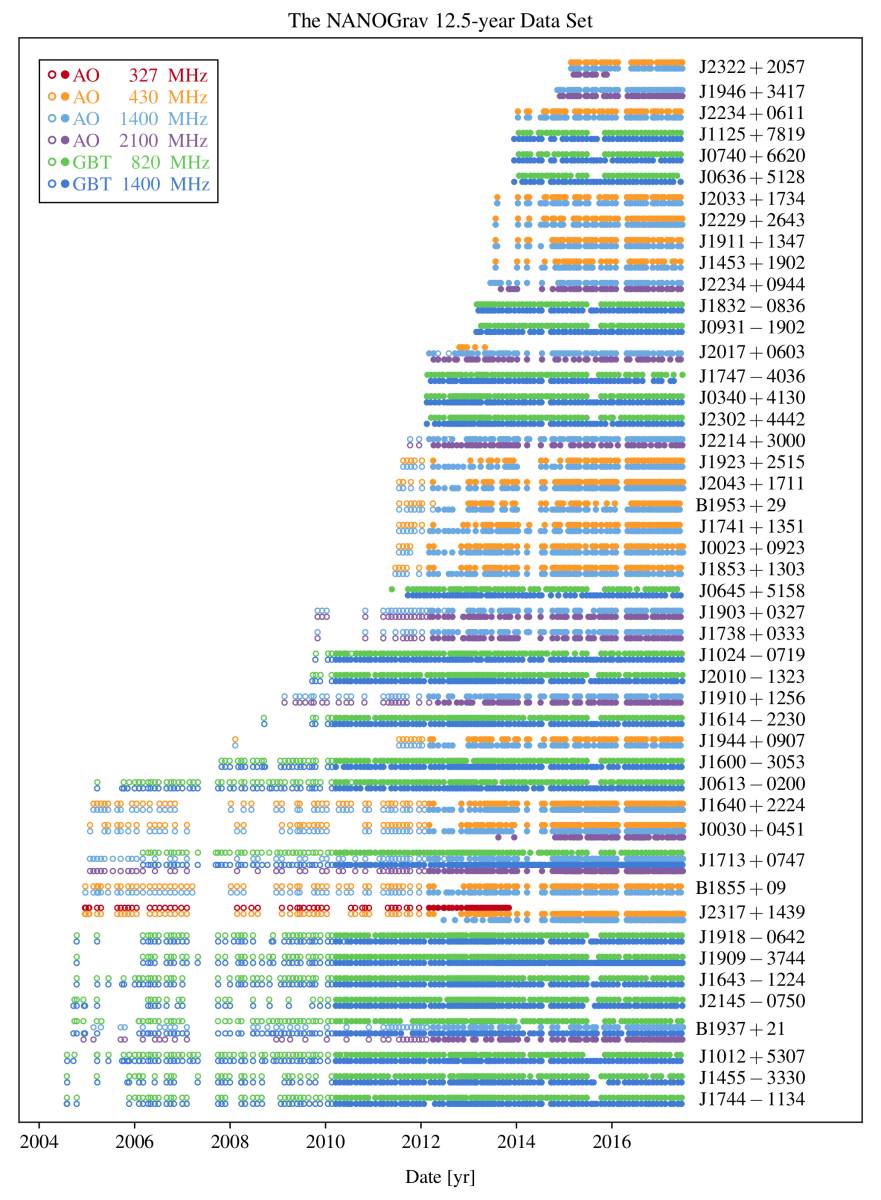# Dataset Tutorials

The tutorials here show how to search for the gravitational waves in NANOGrav data. They all use ENTERPRISE and its extensions software for which installation instructions are included on the GitHub page associated with each tutorial. Bayesian sampling is done using PTMCMCSampler.

## 12.5 Year Stochastic Background

ImageData time-span and frequency information for the NANOGrav 12.5y dataset.

NANOGrav searched for an isotropic stochastic gravitational-wave background in the NANOGrav 12.5-year dataset and reported its results in The Astrophysical Journal Letters. The tutorials below demonstrate the methods used in that analysis and they reproduce some key figures from the paper. All tutorials and installation instructions can be found on the 12.5 year stochastic analysis tutorials GitHub page.

### Single Pulse GWB Analysis (Google Colab)

This tutorial is meant to be a quick introduction for those who don't have time to run the computations required in the full pulsar timing array (PTA) gravitational wave background (GWB) analysis. We go through the Bayesian analysis of J1909 and J1713. These are two of the longest timed pulsars in the NANOGrav data set. One of the pulsars supports the GWB, while the other does not.

### White Noise Analysis of a Single Pulsar (Google Colab)

Before beginning the large data analyses, we begin by analyzing the noise in each pulsar using a Bayesian framework. We run a model with white noise and intrinsic red noise to find out the most likely values for white noise parameters. By saving these values in a noise file, we may set the white noise as constant in the isotropic stochastic gravitational wave background analysis using these noisefiles. This tutorial contains a single pulsar white noise run both in long form and also using enterprise extensions to do everything in a few lines. This tutorial will take a few hours to complete due to the large time requirement to sample the posteriors.

### PTA Stochastic Background Analysis (Google Colab)

In this tutorial, we work through the Bayesian isotropic gravitational wave background analysis of a subset of pulsars in the NANOGrav data set (those timed for more than 6 years). After going through a generalized process of the single pulsar GWB analysis, we show how to compare models and compute Bayes factors. Figures 1, 2, and part of 3 of the 12.5 year stochastic background paper are reproduced in this notebook. This tutorial will take a few hours to complete due to the large time requirement for sampling posteriors.

### Optimal Statistic Analysis (Google Colab)

This tutorial gives an introduction to frequentist methods we can use to look for the stochastic gravitational-wave background. It calculates the optimal statistic using the maximum likelihood noise parameters and also the noise marginalized optimal statistic using the noise parameter posteriors from a Bayesian analysis. It reproduces Figure 4 and 5 of the paper.